# Wiener-Wintner theorem

(Redirected from Wiener–Wintner theorem)
Jump to: navigation, search

Wiener–Wintner ergodic theorem

A strengthening of the pointwise ergodic theorem (cf. also Ergodic theory) announced in [a21] and stating that if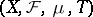is a dynamical system, then givenone can find a set of full measuresuch that forin this set the averages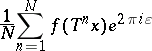converge for all real numbers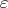. In other words, the set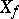"works" for an uncountable number of. This introduces into ergodic theory the study of general phenomena in which sampling is "good" for an uncountable number of systems. Since [a21], several proofs of the "Wiener–Wintner theorem" have appeared (e.g., see [a11] for a spectral path and [a14] for a path using the notion of disjointness in [a13]).

## Uniform Wiener–Wintner theorem and Kronecker factor.

Foran ergodic dynamical system (cf. also Ergodicity), the Kronecker factorofis defined as the closed linear span inof the eigenfunctions of. The orthocomplement of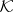can be characterized by the Wiener–Wintner theorem. More precisely, a functionis in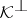if and only if for-a.e. with respect to,This theorem was announced by J. Bourgain [a9]. Other proofs of this result can be found in [a1] and [a15], for instance.

A sequence of scalarsis a good universal weight (for the pointwise ergodic theorem) if the averages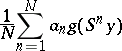converge-a.e. for all dynamical systems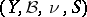and all functions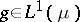. Bourgain's return-time theorem states that given a dynamical systemand a functionin, then for-a.e. with respect to, the sequence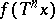is a good universal weight (see [a8]). By applying this result to the irrational rotations on the one-dimensional torus given byand to the function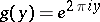, one easily obtains the Wiener–Wintner theorem. Another proof of his result can be found in [a10] and [a19]. Previous partial results can be found in [a11].

## Wiener–Wintner return-time theorem and the Conze–Lesigne algebra.

A natural generalization of the return-time theorem is its Wiener–Wintner version, in which averages of the sequence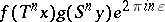are considered. Such a generalization was obtained in [a7] and one of the tools used to prove it was the Conze–Lesigne algebra. This algebra of functions was discovered by J.P. Conze and E. Lesigne [a12] in their study of the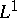norm convergence of the averages(a1)

for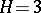. These averages were introduced by H. Furstenberg. (The functionsare in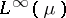. The-norm convergence of (a1) for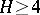is still an open problem (as of 2001).) It is shown in [a7] that the orthocomplement of the Conze–Lesigne factor characterizes those functions for which outside a single null set ofindependent oforone has-a.e.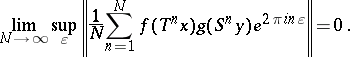Several results related to the ones above can be found in [a2], [a3], [a4], [a16], [a18], [a20], [a17], and [a22]. In [a5] it was shown that many dynamical systems have a Wiener–Wintner property, based on the speed of convergence in the uniform Wiener–Wintner theorem; this allows one to derive the results in [a8] and [a9] for such systems in a much simpler way.